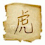## Friday, January 23, 2015

### 52 Things: Number 16: Describe the key generation, signature and verification algorithms for DSA, Schnorr and RSA-FDH.

This is the latest in a series of blog posts to address the list of '52 Things Every PhD Student Should Know To Do Cryptography': a set of questions compiled to give PhD candidates a sense of what they should know by the end of their first year. In this week, we describe the key generation, signing and verification algorithms of DSA, Schnorr and RSA-FDH.

1. DSA
The Digital Signature Scheme (DSA), also known as the Digital Signature Standard (DSS), was proposed by the National Institute of Standards and Technology (NIST) in 1991 . Security of DSA is based on the difficult of computing discrete logarithms. But there is no known proof of its security under a standard assumption (like DL), even in the random oracle model

Domain Parameter Generation
1. Select a prime number $p$, where $2^{L-1}<p<2^L$ and $L$ is a multiple of 64 and $512 \leq L \leq 1024$.
2. Select a prime divisor $q$ of $p-1$, where $2^{159}<q<2^{160}$.
3. Compute a generator $g$ of the subgroup of order $q$: choose a random integer $r$, where $1<r<p-1$ such that $g=r^{(p-1)/q} \ mod \ p$ and $g \neq 1$.
Key Generation
1. Select a random integer $x$, where $0<x<q$.
2. Compute $y = g^x \ mod \ p$.
Then the public key is $y$ and the private key is $x$.

Signing
1. Select a random integer $k$, where $0<k<q$.
2. Compute $r = (g^k \ mod \ p)\ mod \ q$.
3. Compute $s = (h(m)+x\cdot r)\cdot k^{-1}\ mod \ q$, where $h(m)$ is hash of $m$ using SHA-1
The signature on $m$ is the pair $(r, s)$.

Verification
1. Compute $u_1 = h(m) \cdot s^{-1}\ mod \ q$.
2. Compute $u_2 = r \cdot s^{-1}\ mod \ q$.
3. Compute $v = (g^{u_1} \cdot y^{u_2}\ mod \ p)\ mod \ q$.
4. Output $1$ if $v = r$, otherwise output $0$.
Correctness
If $(r,s)$ is a valid signature on $m$, then we have
$v = g^{u_1}\cdot y^{u_2}=g^{h(m)\cdot (h(m)+x\cdot r)^{-1}\cdot k}\cdot {g^{x \cdot r \cdot (h(m)+x\cdot r)^{-1}\cdot k}} \ mod \ p$
$= g^{(h(m)+x\cdot r)\cdot (h(m)+x \cdot r)^{-1}\cdot k}\ mod \ p$
$= g^k \ mod \ p$
Thus the verification succeeds.

2. Schnorr
The Schnorr signature is an important DLP-based signature scheme. It works in any prime order group and its security is proven in the random oracle model under DL assumption .

Domain Parameter Generation
1. Select a prime number $p$.
2. Select a prime divisor $q$ of $p-1$.
3. Select a generator $g$ of the subgroup of order $q$.
Key Generation
1. Select a random integer $x$, where $0<x<q$.
2. Compute $y=g^x \ mod \ p$.
The public key is $y$ and the private key is $x$.

Signing
1. Select a random integer $k$, where $0<x<q$.
2. Compute $a = g^k \ mod \ p$.
3. Compute $r = h(m \| a)$, where $m$ is the message to be signed and $h:\{0,1\}^* \rightarrow \mathcal{Z}_{q}$ is a hash function.
4. Compute $s = (k + r\cdot x)\ mod \ q$
The signature on $m$ is the pair $(r,s)$.

Verification
1. Compute $v = g^s \cdot y^{-r}\ mod \ p$
2. Output $1$ if $v = r$, otherwise output $0$.
Correctness
If $(r,s)$ is a valid signature on $m$, then we have
$v=g^s\cdot y^{-r}=g^{k+r\cdot x}\cdot g^{-r\cdot x}=g^k=r$
Thus the verification succeeds.

3. RSA-FDH
The RSA-FDH (full domain hash) scheme was introduced by Bellare and Rogaway in . It is a RSA-based signature scheme and follows the hash-then-sign paradigm. It makes use of the hash function (the image size of the hash function equals to RSA modulus) to generate random-looking output for the plain RSA signature scheme. Thus it prevents the algebraic attacks on the plain RSA signature scheme and it is able to sign messages of arbitrary length. But it is hard to create such hash function in practice. RSA-FDH can be proven EU-CMA secure in the random oracle model

Key Generation
1. Select two large primes $p$ and $q$.
2. Compute $N=p\cdot q$.
3. Select a random integer $e$, where $1<e<\phi(N)$, such that $gcd(e,\phi(N))=1$.
4. Compute the integer $d$, where $1<d<\phi(N)$, such that $e\cdot d = 1\ mod \ \phi(N)$.
The public key is $(N,e)$ and the private key is $(d,p,q)$.

Signing
1. Compute $s=h(m)^d\ mod \ N$, where $m$ is the message to be signed and $h:\{0,1\}^* \rightarrow \mathcal{Z}_N$ is a hash function.
The signature on $m$ is $s$.

Verification
1. Output $1$ if $s^e = h(m)\ mod \ N$, otherwise output $0$.
Correctness
If $s$ is a valid signature on $m$, then we have
$s^e=h(m)^{d\cdot e} mod \ N=h(m)\ mod \ N$
Thus the verification succeeds.

http://nvlpubs.nist.gov/nistpubs/FIPS/NIST.FIPS.186-4.pdf
http://eprint.iacr.org/2012/029.pdf
http://web.cs.ucdavis.edu/~rogaway/papers/exact.pdf

1.1.